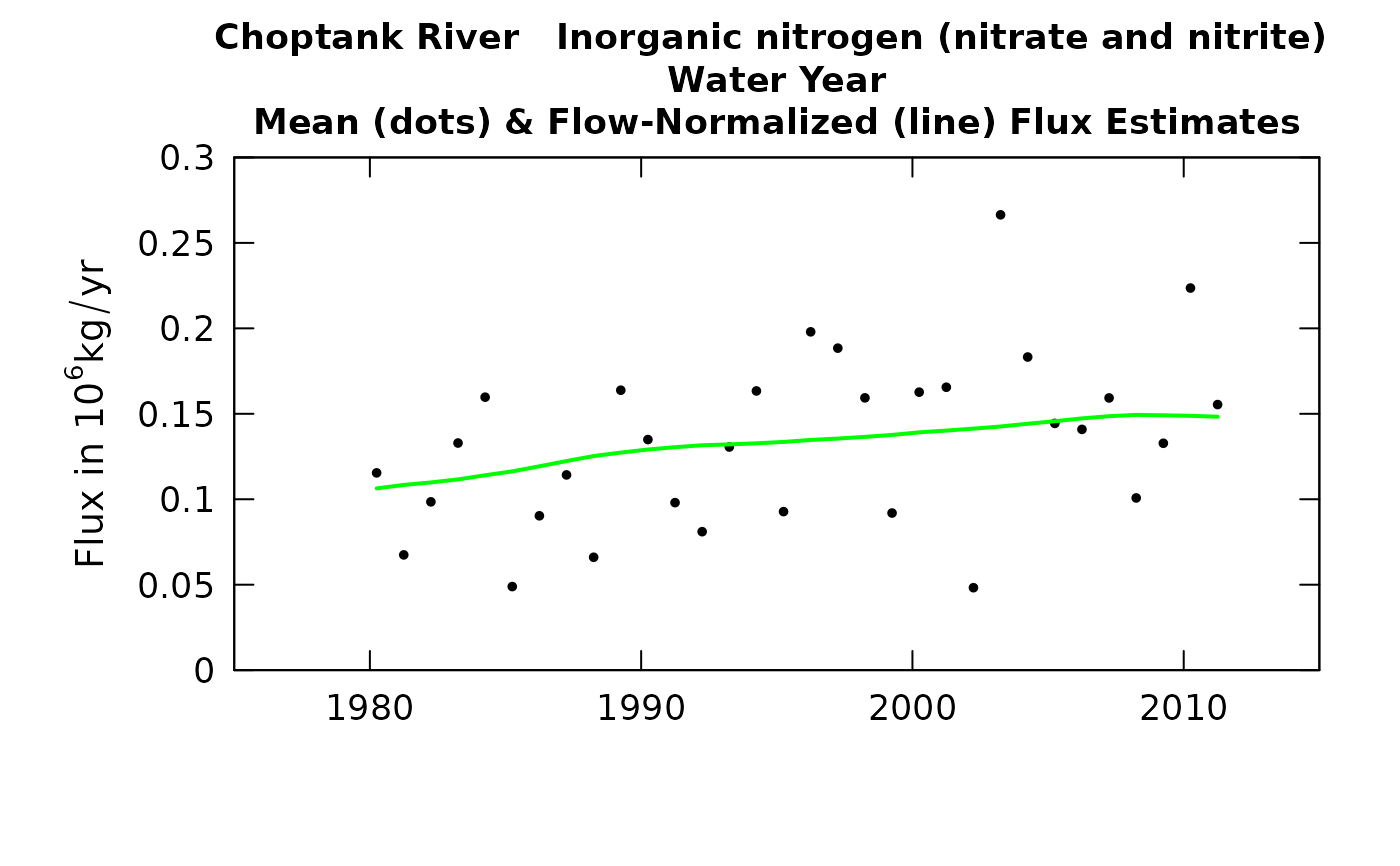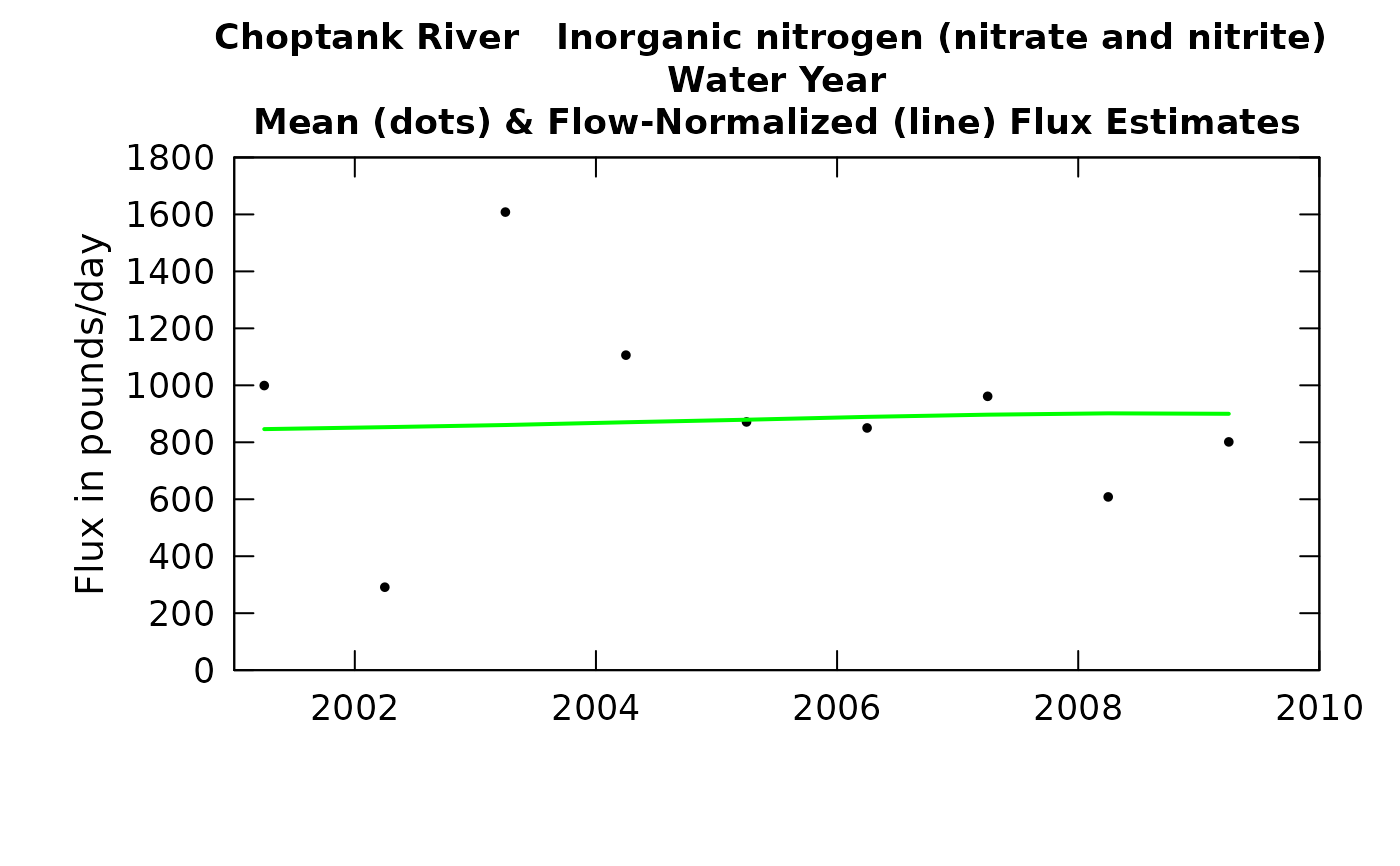The annual results reported are for a specified "period of analysis" which can be an entire water year, a calendar, a season or even an individual month. The user specifies this period of analysis in the call to setupYears. Values plotted express a flux rate, such as thousand kg per year For a period of analysis that is less than a year, this does not equal the mass transported over the period of analysis

Although there are a lot of optional arguments to this function, most are set to a logical default.

Data come from named list (eList), which contains a Daily dataframe with the daily flow data, and an INFO dataframe with metadata.

## Usage

plotFluxHist(eList, yearStart = NA, yearEnd = NA, fluxUnit = 9,
fluxMax = NA, printTitle = TRUE, usgsStyle = FALSE,
plotFlowNorm = TRUE, plotAnnual = TRUE, plotGenFlux = FALSE,
tinyPlot = FALSE, col = "black", col.pred = "green", col.gen = "red",
cex = 0.8, cex.axis = 1.1, cex.main = 1.1, lwd = 2,
customPar = FALSE, ...)

## Arguments

eList

named list with at least the Daily and INFO dataframes

yearStart

numeric is the calendar year containing the first estimated annual value to be plotted, default is NA (which allows it to be set automatically by the data)

yearEnd

numeric is the calendar year just after the last estimated annual value to be plotted, default is NA (which allows it to be set automatically by the data)

fluxUnit

number representing entry in pre-defined fluxUnit class array. printFluxUnitCheatSheet

fluxMax

number specifying the maximum value to be used on the vertical axis, default is NA (which allows it to be set automatically by the data)

printTitle

logical variable if TRUE title is printed, if FALSE title is not printed (this is best for a multi-plot figure)

usgsStyle

logical option to use USGS style guidelines. Setting this option to TRUE does NOT guarantee USGS compliance. It will only change automatically generated labels.

plotFlowNorm

logical variable if TRUE the flow normalized line is plotted, if FALSE not plotted

plotAnnual

logical variable if TRUE annual flux points are plotted, if FALSE not plotted

plotGenFlux

logical variable. If TRUE, annual flux points from WRTDSKalman output are plotted, if FALSE the generalized flux is not plotted.

tinyPlot

logical variable, if TRUE plot is designed to be plotted small, as a part of a multipart figure, default is FALSE

col

color of points on plot, see ?par 'Color Specification'

col.pred

color of flow normalized line on plot, see ?par 'Color Specification'

col.gen

color of points for WRTDS_K output on plot, see ?par 'Color Specification'

cex

numerical value giving the amount by which plotting symbols should be magnified

cex.axis

magnification to be used for axis annotation relative to the current setting of cex

cex.main

magnification to be used for main titles relative to the current setting of cex

lwd

number line width

customPar

logical defaults to FALSE. If TRUE, par() should be set by user before calling this function (for example, adjusting margins with par(mar=c(5,5,5,5))). If customPar FALSE, EGRET chooses the best margins depending on tinyPlot.

...

arbitrary graphical parameters that will be passed to genericEGRETDotPlot function (see ?par for options)

setupYears

## Examples

yearStart <- 2001
yearEnd <- 2010
eList <- Choptank_eList
# Water year:
# \donttest{
plotFluxHist(eList)plotFluxHist(eList, yearStart, yearEnd, fluxUnit = 1)plotFluxHist(eList, yearStart, yearEnd, fluxUnit = 'kgDay')# }